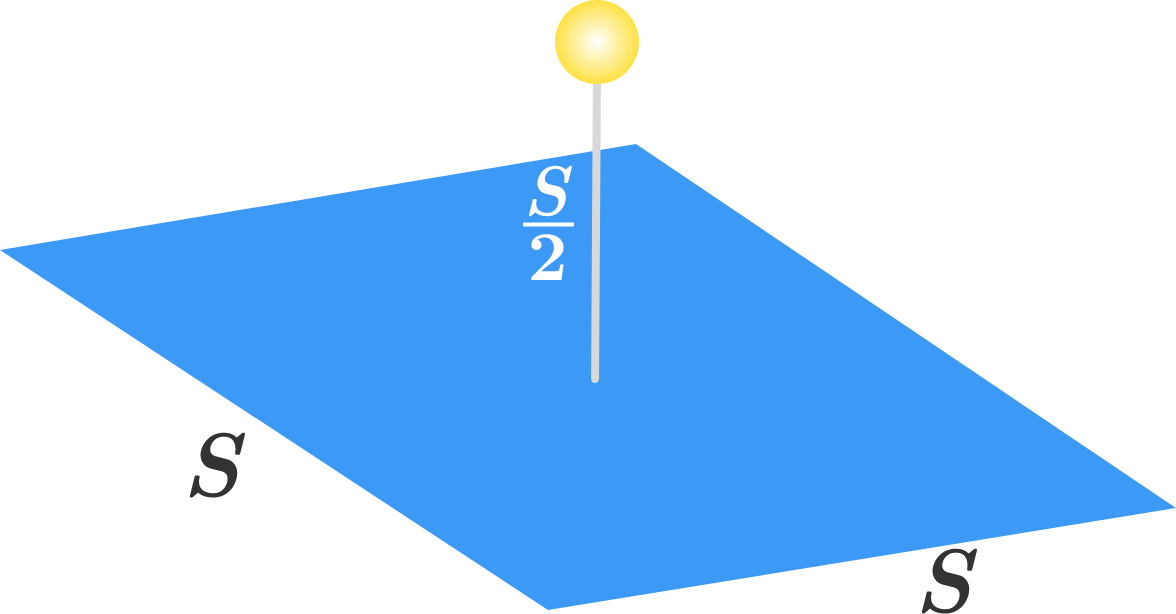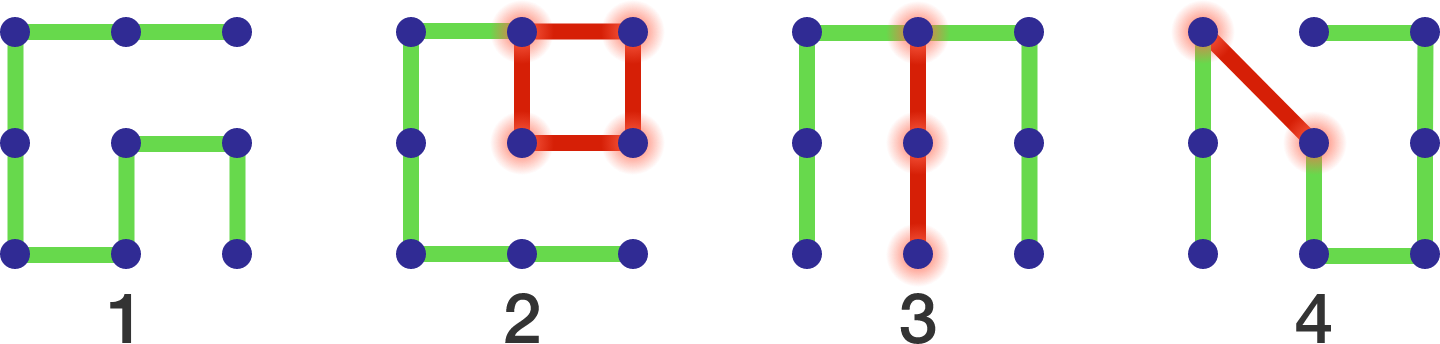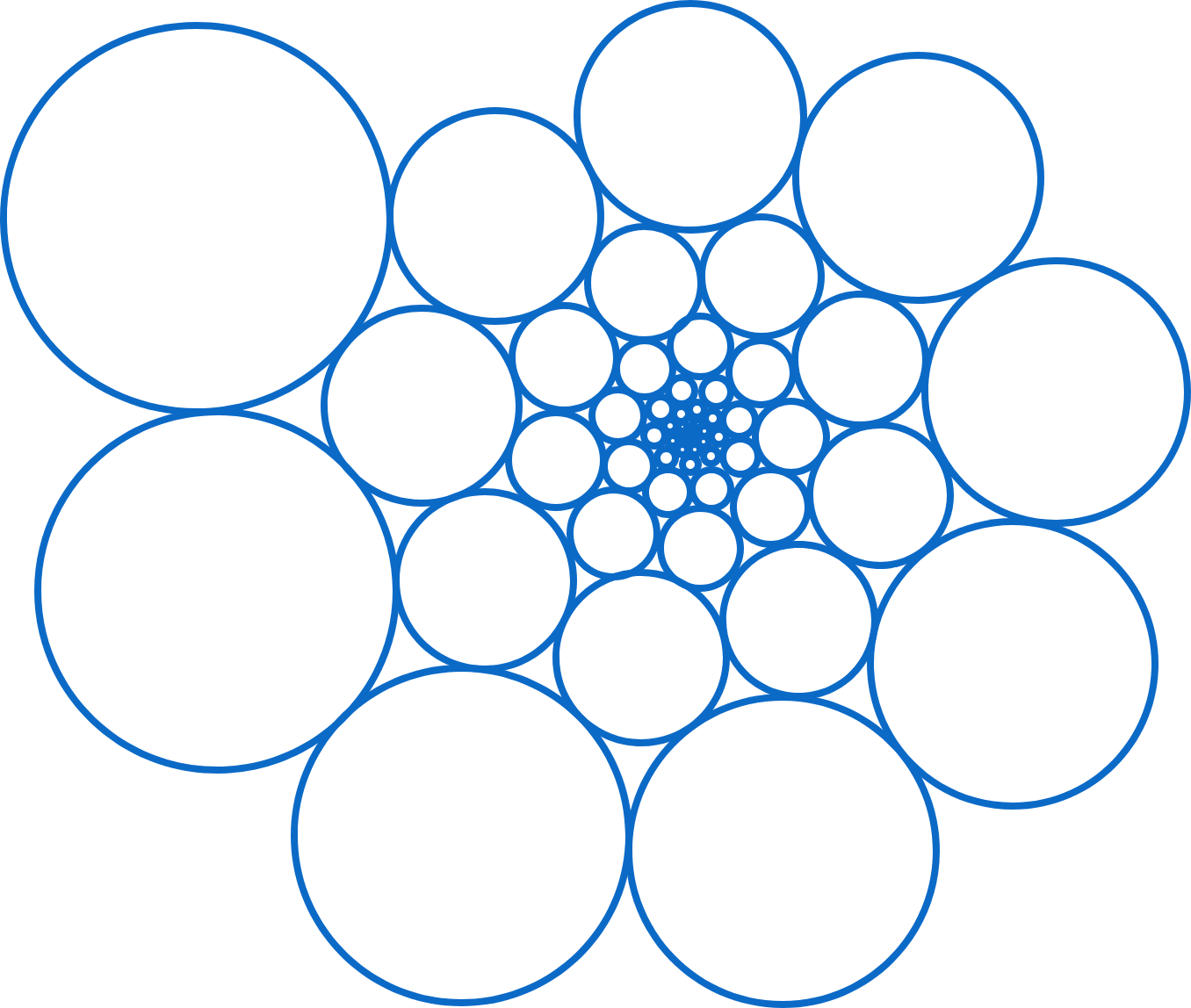# Problems of the Week

Contribute a problem

In the $xyz$-coordinate system, a thin solid square of side length $S$ lies in the $xy$-plane with its center at the origin. An isotropic light source is positioned at $(x,y,z) = \left(0,0,\frac{S}{2}\right)$.

What percentage of the light energy is incident on the square $($to one decimal place; i.e., $66\frac{2}{3}\%$ would be entered as 66.7$)?$$m^3-n^3+mn(m-n)=315^9$

Find the number of solutions $(m, n)$ to the equation above, where $m$ and $n$ are both positive rational numbers and their difference is an integer.

In the pattern lock system below,

• all of the 9 nodes have to be connected without any cycle, and
• any connection using one or more diagonal edges is not allowed.

For example, pattern #1 below is good.Pattern #2 is invalid because it includes a cycle, as indicated by the red square. Pattern #3 is invalid because it contains an implicit cycle, i.e. the red segment must be traveled twice to go from the lower left corner to the lower right corner. Pattern #4 is invalid because a diagonal edge is used to connect 2 nodes.

So, how many different patterns can be generated for this system?

Bonus: What if using diagonal edges is allowed while intersections between 2 or more diagonal edges are prohibited. Can you find the number of different patterns in this case?

Let $x,y,z$ be positive numbers such that $x+y+z=2$ and $xy+yz+zx=1$. Given that $\large x^{20.17}+y^{20.17}+z^{20.17}$ achieves its maximum value when $(x,y,z)=(X,Y,Z)$, and that $XYZ=\frac{a}{b},$ where $a$ and $b$ are coprime positive integers, find $a+b$.

An infinity of circles covers part of the plane in a fractal spiral. As the figure below shows, there is one largest circle, which we'll call the first one, followed by others whose radii are in geometric progression. The $9^\text{th}$ and $10^\text{th}$ circles are tangential to the first circle.

Let $A$ be the total area of all the circles, and $B$ the total area of the interstitial spaces formed by these circles. What is $\left \lfloor 1000 \times \frac{ A } { A+B} \right \rfloor$?Bonus Question: Does "circle area density on the infinite plane" always has a meaning? For a regular array of circles on the infinite plane, it does. When does it fail to have a meaning? Consider the case of "mass density" of the universe, for example. Does that always necessarily have a meaning?

×